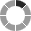# Almont District Library

Loading palette preview# Math Fun

Math Advantage
Divided by grade level (k-8). Build dinosaurs and join Dr. Gee in the 3-D lab. This site is filled with Shockwave games that will make math exciting and fun.

Dr. Math
Need help finding that formula for geometry, or assistance with adding and subtracting fractions? Dr. Math is here to help.

Basket Math Interactive
The ball's in your court, the heat is on, the shot is yours. Solve the math problem to shoot and score.

Explore Your Knowledge
Test your knowledge in math and science and see how you score compared to other students from around the world.

Create a Graph
Use this site to quickly and easily create an area, bar, line, or pie graph. Just simply type in the numbers and titles and you have it.

Math League Help Topics
A great resource for finding math equations and operations and provides examples to see how they work.

Math Dictionary
How exactly do you use an abacus? How long is a fortnight? What exactly is a leap year? … this and more.

Try out your Multiplication Tables
Tables Practice Tests - Practice your timetables. Choose from 2's to 10's.

Math Puzzles
Read Dr. Math's answers to students' tough story problems or ask him one of your own.

Mad Math
You choose the type of problems you want to do (add, subtract, multiply, divide). Many options make this site a helpful tool in practicing math.